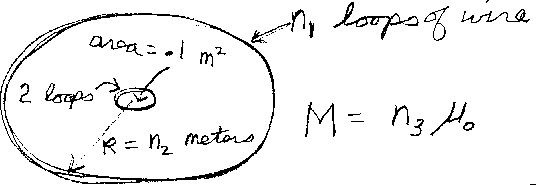Problem C6: In the figure above there are two concentric circular loops of wire that lie in the same plane. The larger loop has n1 turns of wire, and has a radius of n2 meters. The smaller loop has two loops of wire, and an area of 0.1 m2. What is the mutual inductance M of the two wires? If M = n3 μ0, what is n3?n1 = n2 = Input n3:
If you are currently in my class, you can record your grade by entering your name and student ID number (without the leading zeros) below and clicking on "record grade".
 First Name = Last Name = ID = Problem: